# The maximal rank of an elliptic curve as a function of its conductor

Many people believe that ranks of elliptic curves are unbounded. If so, what is the maximal rank of an elliptic curve as a function of its conductor? This question is related to the maximal size of the argument of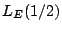for the (normalized) L-function associated with an elliptic curve E. (See the article on the maximal size of $S(T)$.)

If one were to make a guess, the two possibilities which may seem most natural are that the maximal rank of a curve of conductor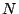is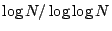(an upper bound which is implied by the Riemann Hypothesis) or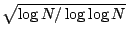(inspired by omega-results for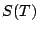).

In the function field case the answer is the larger of these two as recent work of Ulmer [ arXiv:math.NT/0109163] shows. Ulmer conjectures that the larger bound is occasionally achieved for elliptic curves over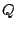.

An elementary version of this problem was formulated by Penney and Pomerance ([MR 51 #12862] and [MR 51 #12861]) for curves of the form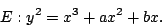Define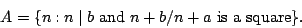Then the rank of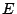is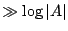. For example, if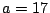and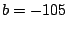, then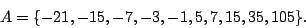Let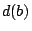be the number of divisors of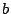. Then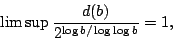so that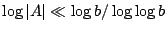. Can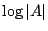be as large as this?

Nick Katz suggests the following problem:

Prove or Disprove: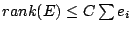, where the conductor ofis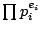, and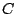is the maximal rank of an elliptic curve with prime conductor.

It is conjectured by Brumer and Silverman [ MR 97e:11062] that the number of of elliptic curves of prime conductor is infinite. So it is not clear that the constant'' in the above problem is finite.

Back to the main index for L-functions and Random Matrix Theory.